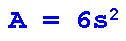HOME

This page shows you how to use the formula for the Total Area of a Cube.

Instructions:

Click on the Example sheet, the Exercise sheet and the Answers sheet in order to view and print them.

Watch the video as many times as you wish.

Use the Example sheet as a model to help you complete the Exercise sheet.

Use the Answers sheet to correct your work.

Video #1: Using the Total Area of a Cube Formula:

Video #2: Why We DON'T Need to Memorize the Total Area of a Cube Formula:

Here is the formula for Total Area of a Cube: